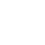Learning through Deterministic Assignment of Hidden Parameter# Learning through Deterministic Assignment of Hidden Parameter

63 views
#WCCI 2014 #Invited Lecture #Zongben Xu #Xian Jiaotong University

In this talk we propose a new TSL scheme: the learning through deterministic assignment of hidden parameters (LDHP). Borrowed from an approximate solution of the classical hard sphere problem and by applying the homeomorphism principle in mathematics, we suggest to deterministically take the hidden parameters as the tensor product of the minimal Riesz energy points on sphere and the best packing points in an interval. We theoretically show that with such deterministic assignment of hidden parameters, LDHP almost shares the same generalization performance with that of OSL, i.e., it does not degrade the generalization capability of OSL. Thus LDHP provides a very effective way of overcoming both the high computation burden of OSL and the uncertainty difficulty in LRHP. We present a series of simulation and application examples to support such advantages and outperformance of LDHP, as compared with the typical OSL algorithm -- Support Vector Regression (SVR) and the typical LRHP algorithm -- Extreme Learning Machine (ELM).

In this talk we propose a new TSL scheme: the learning through deterministic assignment of hidden parameters (LDHP). Borrowed from an approximate solution of the classical hard sphere problem and by applying the homeomorphism principle in mathematics, we suggest to deterministically take the hidden parameters as the tensor product of the minimal Riesz energy points on sphere and the best packing points in an interval. We theoretically show that with such deterministic assignment of hidden parameters, LDHP almost shares the same generalization performance with that of OSL, i.e., it does not degrade the generalization capability of OSL. Thus LDHP provides a very effective way of overcoming both the high computation burden of OSL and the uncertainty difficulty in LRHP. We present a series of simulation and application examples to support such advantages and outperformance of LDHP, as compared with the typical OSL algorithm -- Support Vector Regression (SVR) and the typical LRHP algorithm -- Extreme Learning Machine (ELM).